Homework Help Question & Answers

# 5) If a couple plans to have eleven children, how many gender sequences are possuble A)...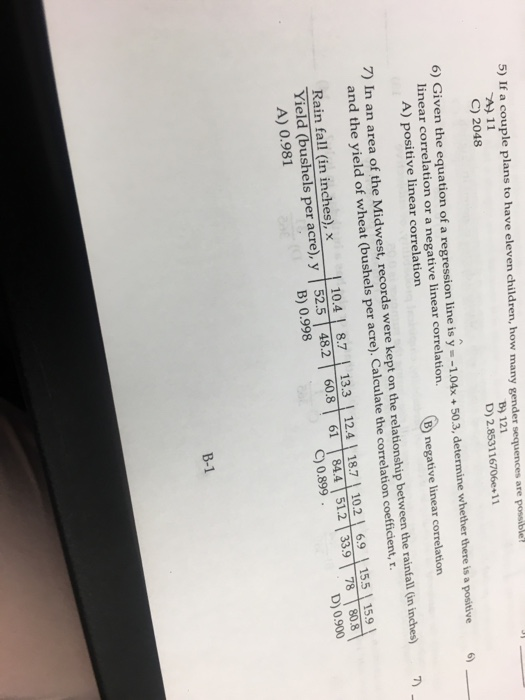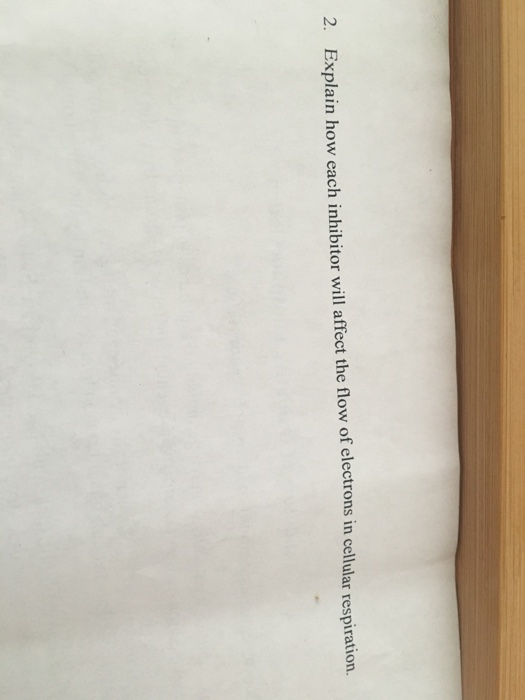5) If a couple plans to have eleven children, how many gender sequences are possuble A) 11 C) 2048 B) 121 D) 2.853116706e 11 6) Given the equation of a regression line is y -1.04x 50.3, determine whether there is a positive 6 inear correlation or a negative linear correlation. A) positive linear correlation B negative linear correlation 7) In an area of the Midwest, records were kept on the relationship between the rainfall (in inches) and the yield of wheat (bushels per acre). Calculate the correlation coefficient, r Rain fall (in inches), x 10.4 8.7 13.3 12.4 18.7 10.2 69 155 15.9 Yield (bushels per acre), y 52.5 482 608 61 84.4 512 33978808 C) 0.899 D) 0.900 B) 0.998 A) 0.981 B-1
2 Esphi bow ec inn

#### Homework Answers

Answer #1

5)

If a couple plans to have eleven children.

Number of gender sequence are possible = 2^11 = 2048

C) 2048

6)

The regression equation is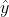= - 1.04x + 50.3

Slope = - 1.04

Slope is negative so the correlation also negative.

The correct option is

B) Negative linear correlation.

7)

We have to find the correlation coefficient ( r ) .

Formula is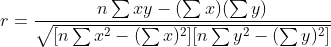n = 9

 x y xy x^2 y^2 10.4 52.5 546 108.16 2756.25 8.7 48.2 419.34 75.69 2323.24 13.3 60.8 808.64 176.89 3696.64 12.4 61 756.4 153.76 3721 18.7 84.4 1578.28 349.69 7123.36 10.2 51.2 522.24 104.04 2621.44 6.9 33.9 233.91 47.61 1149.21 15.5 78 1209 240.25 6084 15.9 80.8 1284.72 252.81 6528.64 Total 112 550.8 7358.53 1508.9 36003.78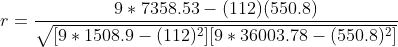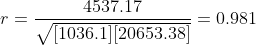A) 0.981

--------------------------------------------------------------------------------

For the remaining question please repost!

Know the answer?
Your Answer:

#### Post as a guest

Your Name:

What's your source?

#### Earn Coin

Coins can be redeemed for fabulous gifts.

Not the answer you're looking for? Ask your own homework help question. Our experts will answer your question WITHIN MINUTES for Free.
Similar Homework Help Questions
• ### In an area of the Great Plains, records were kept on the relationship between the rainfall...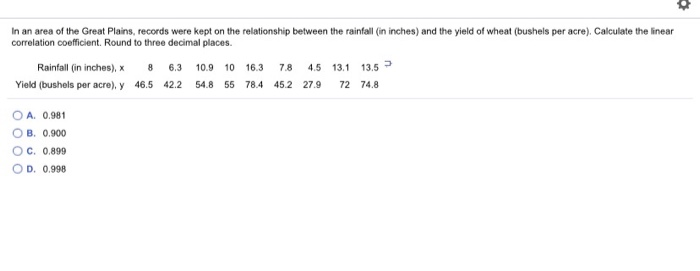In an area of the Great Plains, records were kept on the relationship between the rainfall (in inches) and the yield of wheat (bushels per acre). Calculate the linear correlation coefficient. Round to three decimal places. Rainfall (in inches), 8 6.3 10.9 10 16.3 78 4.5 13.1 13.5 = Yield (bushels per acre), y 46.5 42.2 54.8 55 78.4 45.2 27.9 72 748 O A. 0.981 B. 0.900 OC 0.899 D. 0.998

Free Homework App

Scan Your Homework
to Get Instant Free Answers
Need Online Homework Help?

Get Answers For Free
Most questions answered within 3 hours.